www . DanYEY . co . uk
 CMOS Logic
Here are some notes on CMOS (complementary metal oxide semiconductor) logic circuits, vaguely inspired by CS2212.

As usual, be aware that they may be incorrect or incomplete.

CMOS logic gates are constructed from MOSFETs. They are assumed to be perfect switches: a 1 on the gate of an n-type allows conduction between the source and drain; a 0 on the gate of a p-type allows conduction.

The CMOS inverter (NOT gate):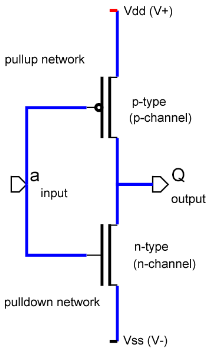Which, in usual symbols, is equivalent to: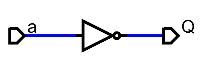The pull-down network pulls the output (Q) low (by allowing flow from the output to Vss), that is, it performs the negation of the function the circuit is supposed to perform. Conversely, the pull-up network pulls the output high (by allowing flow from Vdd to the output). OR functions are produced by having transistors in parallel (electrons could flow through either path). AND functions are produced by placing transistors in series.

A NAND gate is produced as follows:
1. The required function is Q = -(A . B).
2. The pull down network is therefore A . B.
3. Two MOSFETs in series, connected to A and B, perform this function.
4. The pull up function is Q = -(A . B) = -A + -B (by De Morgan's theorem). The pull up function must be in NNF (negation normal form), that is, only variables may be negated.
5. MOSFETs in parallel will do this function nicely.
6. We connect the pull-up MOSFETs directly to A and B, since p-type MOSFETs ordinarily "negate".
7. This gives us:The power dissipated by a CMOS logic gate is P = C.Vdd².f, where C is the capacitance of the load and f is the switching frequency of the circuit.

Another important formula is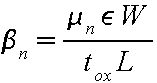where mu is the mobility of the charge carriers, epsilon is the permativity of the substrate, tox is the thickness of the gate oxide, and W and L are the dimensions of the transistor channel.

Layout And Fabrication
CMOS circuits fabricated with various layers of materials (primarily silicon). The MOSFET is constructed as in the diagram (of an n-type) below:The p-type is constructed similarly, except the p-type substrate is replaced with n-type (an n-well), the drain and source implants are replaced with p-type material, and the names drain and source swap over (the names refer to the charge carriers - a p-type's charge carriers are holes, not electrons).

When a voltage is applied to the polysilicon gate, a charge is induced in the channel. The thin oxide insulates the channel from the gate. The charge induced in the channel allows conduction between the drain and source.

The inverter, then, has a cross section like: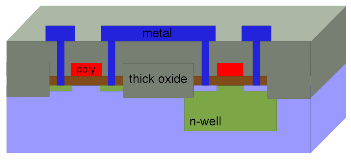And from above (layout view):The thick oxide is used to insulate the transistors from the metal, which is used to form all non-input circuits.

Other Uses Of MOSFETs
A pair of MOSFETs can be used more directly as a switch by connecting two like so:This is called a transmission gate. When a 1 is applied to the control, both conduct whatever logic level is on the input, otherwise, neither transistor conducts (two transistors are used because n-types transfer logic 1s badly, and p-types transfer logic 0s badly).

nMOS logic is an alternative way of making logic functions. Instead of a pull-up network, there is simply a resistor. The output is normally high.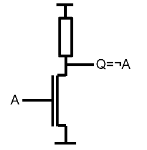Programmable logic devices (PGA/PLA/PAL/ROM/FPGA) can be built using nMOS logic and "floating gate" transistors. This has an extra gate which operates like a normal transistor (providing an invert function) until a high voltage is passed through the channel, which affects the floating gate such that it no longer allows conduction (until it is erased with UV). The floating gate stops providing an inversion, so, a logic 1 has effectively been written to the programmable device.